# How to Calculate Year, Month & Days Using VBA in Microsoft Excel 2010

In this article, you will learn how to calculate year, month & days. We will use VBA code to create UDF function for calculation.

Click on Developer tab

From Code group, select Visual Basic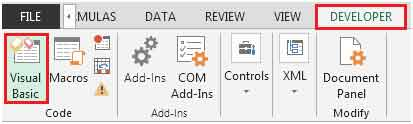Click on Insert, and then Module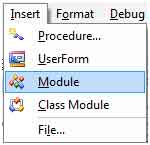This will create a new module.

Enter the following code in the Module

Function dDATEDIF(Start_Date As Date, End_Date As Date, Unit As String) As String

xlDATEDIF = DateDiff(Unit, Start_Date, End_Date)

End Function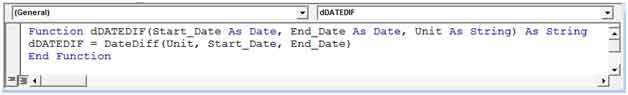The new UDF formula is created with name dDATEDIF

There are three parameters:

Start_Date: The days from which the period begins.

End_Date: It is the last date of the period that you wish to calculate.

Unit: It specifies the interval by which you want the difference. Here, the unit accepts the following values.

To find the number of days, the formula would be =dDATEDIF(A2,B2,"d"). Refer below snapshot: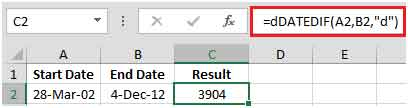To find the number of years, the formula would be =dDATEDIF(A2,B2,"yyyy"). Refer below snapshot: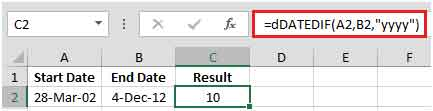To get other outputs like month, quarter, etc., refer below snapshot, which can be used as a Unit (3rd parameter), and the result will get updated.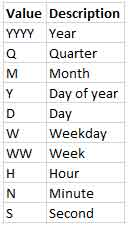In this way you can get to know the all the time values that you are interested in.

Terms and Conditions of use

The applications/code on this site are distributed as is and without warranties or liability. In no event shall the owner of the copyrights, or the authors of the applications/code be liable for any loss of profit, any problems or any damage resulting from the use or evaluation of the applications/code.